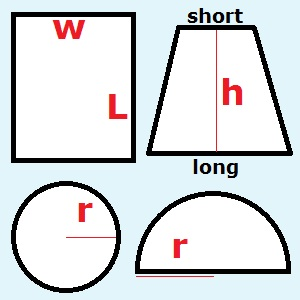# How Many Bricks Do I Need to Pave a Patio or Driveway?Patio or Driveway Shape rectangle trapezoid circle half-circle Patio Width (rectangle) ft. Patio Length (rectangle) ft. Patio Height (trapezoid) ft. Patio Long Side (trapezoid) ft. Patio Short Side (trapezoid) ft. Patio Radius(circle and half-circle) ft. Brick Width in. Brick Length in. Brick Spacing in. Number of Bricks Needed = Extra Bricks Needed =

Brick patios can bring out the best features of your landscaping and dramatically increase the value of your home. Paving a patio or driveway with brick may be more expensive and time consuming than paving with concrete or laying slate tile. However, a brick patio is less likely to develop unsightly cracks, and nothing matches the elegant look of a well-laid brick surface. The number of bricks needed to pave a driveway or patio depends on the area of the patio, the area of the bricks, and the length of spacing between the bricks. You can use the brick calculator on the left to estimate the total number of bricks needed to pave an area outdoors.

First determine the shape of the surface. Most driveways and patios are either rectangles, trapezoids, circles, or half-circles. If your driveway is rectangular, select 'rectangle,' and fill in the width and length in feet. If your driveway is a trapezoid shape, select 'trapezoid,' and fill in the height, long side, and short side. For circular and semi-circular patios, select either 'circle' or 'half-circle,' and fill in the radius.

Next, enter the brick width and length in inches. Also input the length of spacing in inches. Do not worry about converting inches to feet to make the calculations correct; the calculator does this for you.

Hit 'Calculate,' and the calculator will tell you how many bricks you need to complete the paving project.

For example, suppose your patio is a 16 foot by 10 rectangle, each brick is 4 inches by 8 inches, and the spacing is 0.5 inches between each brick. Then the total number of bricks you will need is about 602. The calculator also estimates the number of spare bricks by taking 5% of the total, so you should buy 30 more bricks to be on the safe side.

You can also apply the formula below and calculate the number of bricks by hand. If A is the area of the patio in square feet, W is the length of the brick in inches, L is the length of the brick inches, and S is the spacing in inches, then the number of bricks you need is

[144*A]/[(W+S)*(L+S)].

To calculate the number of extra bricks needed, use the formula

[7.2*A]/[(W+S)*(L+S)].